## Example Questions

← Previous 1

### Example Question #1 : How To Find Proportion

Max will be age X in 5 years. How old was he 4 years ago?

X – 5

X – 4

X +5

X – 9

X – 9

Explanation:

Max will be X in 5 years. So today he is X -5 and 4 years ago he was (X-5)  - 4, that is X – 9.  It always helps to put numbers into the equations. Example: Max will be 12 in 5 years. How old was he 4 years ago? So today Max is 12 – 5 = 7.  Therefore, four years ago he was 7 -4 =3. Which is 12-5-4 =12-9 = 3

### Example Question #2 : How To Find Proportion

If a pizza pie that has 16 slices and costs $12.00 is to be shared among 8 friends, how much should each person contribute? Possible Answers:$0.75

$1.50$1.75

$2 Correct answer:$1.50

Explanation:

It is $12 divided by 8 which is$1.50. The number of slices in the pizza pie is not relevant in this question.

### Example Question #3 : How To Find Proportion

In a school relay race, every 5-man team must sprint 110 yards total.  However, Jim’s team is short a person.  Thus, Jim must run two sections for his team.  How far does Jim have to run?

45.4 yards

110 yards

44 yards

22 yards

27.5 yards

44 yards

Explanation:

We first find out how much each person must run.  Thus we take 110/5 to get 22 yards.  However, Jim must run twice the amount of a normal competitor, so we multiply by 2 to get 44 yards.

### Example Question #4 : How To Find Proportion

A building that is 30 feet tall casts a shadow that is 50 feet long. If another building casts a shadow that is 100 feet long, how tall is the building?

600 feet

6 feet

167 feet

60 feet

1670 feet

60 feet

Explanation:

This problem can be set up as a proportion: 30 feet/x feet = 50 feet/100 feet. To solve, we simply cross multiply: (30 feet * 100 feet) = (50 feet * x feet). Thus, 3000 feet = 50x feet. To solve for x, divide each side by 50. Therefore, = 60 feet.  If you got 167 feet, you may have set up the proportion incorrectly by mixing up the height of the building with the length of the shadow. If you got 6 feet or 600 feet, you may have made a computational error. If you got 1670 feet, you may have set the proportion up incorrectly and made a computational error.

### Example Question #5 : How To Find Proportion

If a bicyclist can bike 24 miles per hour, how far (in miles) can he travel in 2 minutes, assuming he bikes at a constant speed (answer rounded to the nearest tenth)?

0.75

0.5

0.8

2.0

1.0

0.8

Explanation:

0.8 mile.  Using some conversions:  ( (24mi/1hr)*(1hr/60min)*2min = 0.8 mile

### Example Question #6 : How To Find Proportion

If 10,000 lbs of cement makes 85,000 lbs of concrete, how many pounds of concrete can be made with 3,000 pounds of cement?

363

12,230

10,000

30,000

25,500

25,500

Explanation:

25,500 lbs of concrete. Setting up a ratio with x representing the number of pounds concrete the 3,000 of cement produces, we obtain the relation: (10,000/85,000) = (3,000/x), x = 25,500 pounds of concrete.

### Example Question #7 : How To Find Proportion

There are 150 students in a lecture hall class in college. 12% of the students received an A. 20 students received a B. Twice the number of students who earned an A received a C. The remainder of the students received a D. Which grade did the students receive more than any other?

Cannot be determined.

The students who got C's

The students who got B's

The students who got A's.

The students who got D's.

The students who got D's.

Explanation:

First find 12% of 150, so 0.12 * 150 = 18 students received an A.

20 students received a B, and 36 students received a C (double the A's).

To find the number of D-grades, all we have to do is subtract these from the total (since there were no grades of F),

Thus: 150 – 18 – 20 – 36 = 76 students who received a D in the course, which is the most common grade.

### Example Question #8 : How To Find Proportion

A brownie recipes calls for a 1:5 ratio of water to brownie mix. If you need 90 cups of brownie mix, how much water do you need?

450 cups

18 cups

6 cups

25 cups

36 cups

18 cups

Explanation:

First set up a proportion, 1/5 = x/90, then solve for x: 5x = 90 → x = 18 cups.

### Example Question #9 : How To Find Proportion

If a 12 oz can of lemonade has 75 calories; how many calories are in an 8 oz can of lemonade?

50

70

55

65

60

50

Explanation:

A proportion is a statement of equality between two fractions or two ratios. Set up a proportion between the size of the drink and the calories. To solve a proportion cross multiply and solve the resulting equation.

75/12 = x/8 → 150/24 = 3x/24 → 50/8 = x/8 → x = 50

### Example Question #91 : Fractions

If there are 75 calories in a 6 oz glass of juice, how many calories will there be in an 8 oz glass?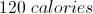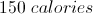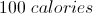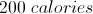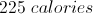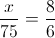where= calories.
We cross multiply to get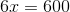, so there will be 100 calories in 8 oz of juice.